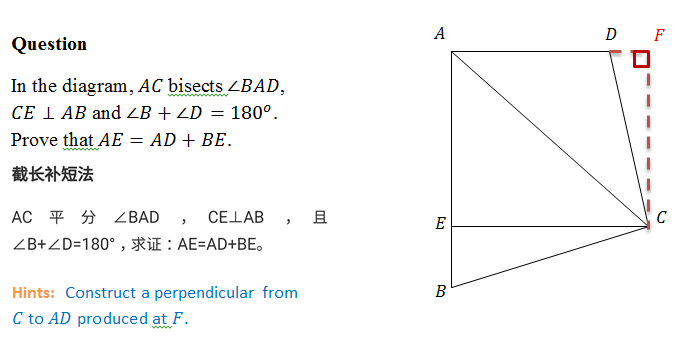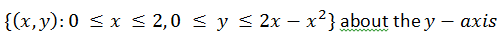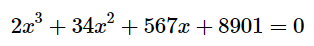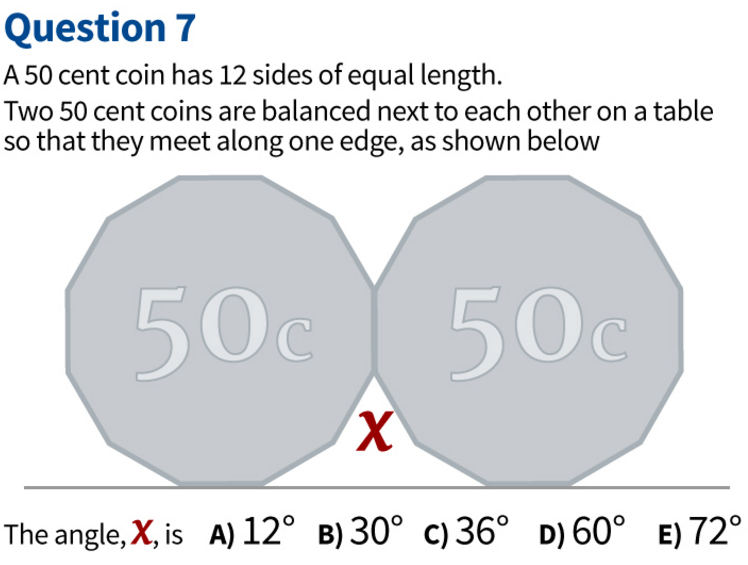## Forum Posts

tosophiechen
Oct 20, 2017
In Solve Me if You can!
Another problem is on congruency of triangles to challenge the motivated students in plane geometry.0
0
5
tosophiechen
Jul 08, 2017
In Challenges Wanted!0
0
27
tosophiechen
May 04, 2017
In Challenges Wanted!
This question was presented by Johnny from our Ext. 2 maths class (2017) via wechat! : ) Use the method of slicing to find the volume of the solid obtained by rotating the region.1
1
47
tosophiechen
May 04, 2017
In Solve Me if You can!
Suppose that we are told that four numbers a,b,c,d lie between -5 and 5. Suppose also that the numbers are constrained so that 5 < a+b < 10 and -10 < c+d < -5 Given this information, what can you deduce about these inequalities? ?? < a+b-c-d < ?? ?? < a-c < ?? ?? < a-c+d-b < ?? ?? < abcd < ?? Did you know ... ? There are many useful general inequalities in mathematics, such as the AM-GM, Cauchy-Schwarz and Jensen's inequalities. These general inequalities are powerful tools which greatly simplify a wide variety of problems in mathematics, in applications from integration to probability via linear algebra.
0
0
4
tosophiechen
May 04, 2017
In Solve Me if You can!
Find a solution to this equation to 1 dp. Are there any others? Did you know ... ? Numerical solution of equations forms an important part of real-world mathematics and mathematics applied to science, where equations are often too complex to be solved exactly. Mathematicians have developed many advanced techniques for the numerical solution and exploration of equations.0
0
10
tosophiechen
May 04, 2017
In Solve Me if You can!
Here it is. Can you solve it?0
0
9